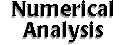[an error occurred while processing this directive] The optimization problem is to find the values of the unknown variables that minimize or maximize the objective functions (affected by the unknown variables) possiblly under certain constraints. Though you might find several routines in Netlib or numerical libraries (as listed in Also Check Out section below) for optimization calculation, the software in this section are usually designed for optimization problems. Search SAL: Optimization   (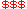Commercial,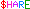Shareware,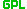GPL) ABACUS-- a software system for the implementation of branch-and-bound algorithms. ACCPM -- solving large scale convex optimization problems with cutting plane method. ASA -- finds the best global fit of a nonlinear non-convex cost-function over a D-dimensional space. Blossom -- a minimum weighted perfect matching solver. bob++ -- C+ classes for sequential or parallel search algorithms for optimization. Cassowary -- a constraint solving toolkit.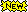COIN -- common optimization interface for operations research. CPLEX-- an optimization software tools for large-scale and mission-critical applications. CSDP -- a library for semidefinite programming. CSPLib -- a problem library for constraints. CUTE -- a suite of Fortran subroutines for linear and nonlinear optimization. DFNLP -- nonlinear least squares optimization. DOC/DOT-- design optimization control software and design optimization tools. DONLP2 -- minimization of a differentiable function with (in)equality constraints. Elastic Net Method for TSP -- demonstration of elastic net method on solving a travelling salesman problem. ELS/GLS -- efficient and global line search programs. EPO-- a universal parameter optimization program based on an evolutionary algorithm. FortMP-- linear, integer and quadratic programming optimization system. FSQP -- software of feasible sequential quadratic programming algorithm. GAOT-- genetic algorithm optimization toolbox for Matlab. GATSS -- a Genetic Algorithm based solver of the Traveling Salesman problem in g++. GENBLIS -- genetic optimization and bootstrapping of linear structures. HOPDM -- solving large scale linear and convex quadratic programming problems. HQP-- a solver for nonlinearly constrained optimization. IAsolver-- Java applet solving systems of non-linear constraints. IBM Optimization Solutions and LibraryIMPS (Intelligent Mathematical Programming System) -- includes ANALYZE, MODLER, RANDMOD. iSight-- combining the power of design optimization, automation and integration for industry. Java Constraint Library -- provide application services for CSP problem. LANCELOT -- Fortran packages for solving large-scale nonlinearly constrained optimization problems. L-BFGS-B -- large-scale bound-constrained or unconstrained optimization. LINDO-- a tool for solving linear, integer, and quadratic programming problems. LINGO-- a modeling package for a diverse variety of problems. LK the program-- implementation of the Lin-Kernighan heuristic for the Traveling Salesman Problem. LMITOOL -- a user-friendly package for LMI optimization. Linear Programming Plug-In for NExS-- provide linear programming facilities in spreadsheet. lp_solve-- mixed integer linear program solver. LOQO-- a system for solving smooth optimization problems. LSNNO -- optimization with nonlinear objective, bound constraints, and linear network constraints. macopt -- a conjugate gradient optimizer written in C/C++. MAXDET -- software for determinant maximization problems. MCF -- a network simplex implementation. MCS -- global optimization by multilevel coordinate search. MEMT -- parameter estimation and prediction for maximum entropy models in discrete domains. MINQ -- general definite and bound constrained indefinite quadratic programming. MOSEK-- solves linear, convex quadratic and general convex mathematical programs. Network Optimization Codes -- codes for matching, shortest paths, and network flow. NLPJOB -- multicriteria optimization. NLPQL -- constrained nonlinear programming. NLPQLB -- nonlinear programming with very many constraints. NP-Completeness -- efficient algorithm designing for hard computational problems. NUOPT-- an add-on optimization software for S-PLUS data analysis. Omuses-- a tool for the optimization of multistage systems. OPBDP -- a Davis-Putnam based enumeration algorithm for linear pseudo-Boolean optimization. OPT++ -- an object-oriented nonlinear optimization library. OptSolve++ -- C++ software components for nonlinear optimization. parSA -- parallel simulated annealing library for optimization problems. PCx -- an interior-point predictor-corrector linear programming package. David Pisinger's optimization codes -- various C programs for optimization. PLAM -- an algebraic modelling system written in Prolog. Pointer-- optimization software which solves difficult nonlinear problems. Porta -- polyhedron representation transformation algorithm. PPBB -- parallelize sequential Branch-and-Bound algorithms for several architectures. pysimplex -- mixed integer linear programming modules for Python. QGraphi -- WYSIWYG graphical software for some linear programming problems. QL -- quadratic programming. SA (Simulated Annealing)-- optimization by modeling potential solutions as electron spins.SADE-- a genetic based optimisation method that uses differential operators. SDPA -- software for semidefinite programming algorithm. SDPHA -- semidefinite programming homogeneous algorithms. SDPMATH -- a Mathematica package for solving Semidefinite Programs. SDPpack -- a package for semidefinite-quadratic-linear programming. SDPSOL -- a parser/solver for semidefinite programs with matrix structure. SDPT3 -- a Matlab software package for semidefinite programming. SOL -- several widely used optimization software such as MINOS, NPSOL, LSSOL. SolvOpt -- a solver for local optimization problems. SoPlex -- a sequential object-oriented simplex class library. SP -- semidefinite programming software in Matlab. space -- the analysis and optimization of computer models or simulations. StarFLIP++-- reusable iterative optimization library for combinatorial problems with fuzzy constraints SYMPHONY -- branch, cut, and price algorithm for solving integer and mixed-integer programs. TENMIN -- unconstrained optimization using tensor methods. TOMLAB-- a general purpose environment of optimization, based on MATLAB. VBCTOOL -- a graphical interface for visualization of branch cut algorithms. VerGO -- global optimization of a real valued function of many variables. YATTS -- a graphical linear programming teaching tool. Also Check Out: COOOL -- tools for writing optimization code and solving optimization problems. ECLiPSe -- a development environment for constraint programming applications. FlexGA-- a genetic algorithm toolbox to implement evolutionary algorithms. GAlib -- a set of C++ genetic algorithm object, with tools to do optimization. GAMS-- a high-level modeling system for mathematical programming problems. Genetic LISREL-- a evolutionary program which estimates LISREL models. LinAlg -- basic linear algebra and optimization C++ classlib. Harwell Subroutine Library-- a suite of Fortran subroutines for scientific computation. INCT -- induced norm control toolbox. NAG C Libraries-- a large collection of algorithmic routines written in C. NAG Fortran 77 Libraries-- Fortran 77 routines for mathematical and statistical computation. NAG FL90plus and fl90 Fortran 90 Libraries-- Fortran 90 libraries. Netlib Repository -- huge software repository. PORT -- a collection of Fortran 77 routines for many traditional areas of mathematical software. PPBB Library -- parallelize sequential Branch-and-Bound algorithms for several architectures. WNLIB -- a C subroutine library that contains numerous tools useful for programming pratice. Other Resources: Constraints Archive http://www.cs.unh.edu/ccc/archive/ List of Useful Optimization Software http://ucsu.colorado.edu/~xu/software.html NEOS Guide Optimization Tree. http://www.mcs.anl.gov/home/otc/Guide/OptWeb/ NEOS Guide: Optimization Software http://www.mcs.anl.gov/home/otc/Guide/SoftwareGuide/index.html Optimization FAQ on Linear and Nonlinear Programming. http://www.mcs.anl.gov/otc/Guide/faq/ RIOT is a new offering for the WWW audience providing interactive educational and research tools for optimization problems. http://ford.ieor.berkeley.edu:80/riot/ A Short Course in Linear Programming: A short course in linear programming (LP) designed for people who already know linear algebra and want to understand what LP is about. http://www-math.cudenver.edu/~hgreenbe/courseware/LPshort/intro.html Decision Tree for Optimization Software http://plato.la.asu.edu/guide.html Global (and Local) Optimization: it is a comprehensive archive of online information on global optimization, and somewhat less comprehensive on local optimization, collected by Arnold Neumaier. http://solon.cma.univie.ac.at/~neum/glopt.html TSPBIB Home Page: This page intends to be a comprehensive listing of papers, source code, preprints, technical reports, etc, available on the Internet about the Traveling Salesman Problem (TSP) and some associated problems. http://www.ing.unlp.edu.ar/cetad/mos/TSPBIB_home.html Mathematical Programming Glossary http://www-math.cudenver.edu/~hgreenbe/glossary/glossary.htmlDiscrete Methods & Related Tools   |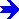Miscellaneous Software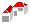SAL Home   |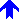Numerical Analysis